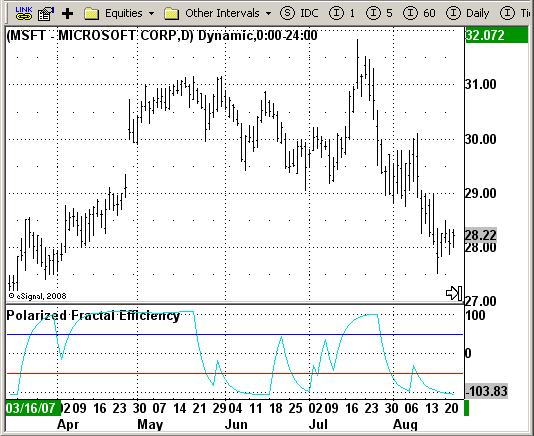# PFE - Polarized Fractal Efficiency

ICE Data Services -

 PFE.efs EFSLibrary - Discussion Board

File Name: PFE.efs

Description:
PFE (Polarized Fractal Efficiency)

Formula Parameters:

Notes:
The Polarized Fractal Efficiency (PFE) indicator measures the efficiency
of price movements by drawing on concepts from fractal geometry and chaos
theory. The more linear and efficient the price movement, the shorter the
distance the prices must travel between two points and thus the more efficient
the price movement.`/*********************************Provided By: eSignal (Copyright c eSignal), a division of Interactive Data Corporation. 2008. All rights reserved. This sample eSignal Formula Script (EFS) is for educational purposes only and may be modified and saved under a new file name. eSignal is not responsible for the functionality once modified. eSignal reserves the right to modify and overwrite this EFS file with each new release.Description: PFE (Polarized Fractal Efficiency)Version: 1.0 10/14/2008Notes: The Polarized Fractal Efficiency (PFE) indicator measures the efficiency of price movements by drawing on concepts from fractal geometry and chaos theory. The more linear and efficient the price movement, the shorter the distance the prices must travel between two points and thus the more efficient the price movement.Formula Parameters: Default:**********************************/var bInit = false;function preMain() { setStudyTitle("Polarized Fractal Efficiency"); setCursorLabelName("EMA", 0); setDefaultBarFgColor(Color.aqua, 0); addBand(50, PS_SOLID, 1, Color.blue); addBand(-50, PS_SOLID, 1, Color.red); }var xEMA = null;var xFracEff = null;function main() {var nBarState = getBarState();var nEMA = 0; if ( bInit == false ) { xFracEff = efsInternal("Calc_FracEff"); xEMA = ema(5, xFracEff) bInit = true; } nEMA = xEMA.getValue(0); if (nEMA == null) return; return nEMA;}function Calc_FracEff(){var PFE = 0;var C2C = 0;var Counter = 0;var FracEff = 0; PFE = Math.sqrt(Math.pow((close(0) - close(-9)),2) + 100); for (Counter = 1; Counter < 10; Counter++) { C2C += Math.sqrt(Math.pow((close(-Counter + 1) - close(-Counter)), 2) + 1); } if ((close(0) - close(-9)) > 0) FracEff = Math.round((PFE / C2C) * 100); else FracEff = Math.round(-(PFE / C2C) * 100); if (FracEff == null) FracEff = 1; return FracEff;}`